Function Repository Resource:

# MonteCarloSample

Sample from a probability density function using the Markov chain Monte Carlo (MCMC) method

Contributed by: Brian Lu
 ResourceFunction["MonteCarloSample"][f,p,n] generates n samples from probability density function f starting from point p. ResourceFunction["MonteCarloSample"][f,{p1,…,pm},n] generates samples starting from an ensemble consisting of m points. ResourceFunction["MonteCarloSample"][f,{dist,m},n] uses probability distribution dist as the prior and samples m points from dist as the initial points. ResourceFunction["MonteCarloSample"][f,p,{n,burn}] generates samples but discards the first burn samples. ResourceFunction["MonteCarloSample"][f,p,{n,burn,i}] generates samples and takes every ith sample.

## Details and Options

The number of arguments that the probability function f takes should match the dimension of the initial points.
The probability function f does not need to be normalized.
When the initial points are specified as {dist,m}, the domain of the prior distribution dist should have the same dimension as the number of arguments of f and should have a probability distribution function (PDF) in symbolic closed form.
The burn specifies the number of samples to be discarded at the beginning of the chain, where thermalization has not been reached, often referred to as "burn-in". The burn can be specified as Automatic, where the length of thermalization steps are automatically determined from the list of probabilities.
The i is a positive integer step size, in which samples are taken. The i can be specified as Automatic, where it is set to be the inverse of the overall acceptance rate with an upper limit of 10.
The following options are supported:
 Method Automatic the MCMC algorithm to use "LogProbability" False whether the probability function f is specified on a log scale "TargetAcceptanceRate" 0.25 the target rate at which proposed points are accepted for certain methods “ListableFunction" False whether the probability function f is listable Parallelization False whether parallelization is used in sampling
Possible settings for Method include:
 "RandomWalk" uses a fixed probability distribution for drawing proposals "AdaptiveMetropolis" use the method of Haario et al. "EMCEE" use the method of Foreman-Mackey et al. "DifferentialEvolution" use the method of ter Braak
For the method "RandomWalk", the following additional suboption is supported:
 "JumpDistribution" the symmetrical probability distribution for drawing proposals
 "InitialScaleMultiplier" 1 the initial scale of the step relative to the default one "RandomWalkScale" 0.1 the absolute scale of steps when random walk is adopted
For the method "EMCEE", the following additional suboptions are supported:
 "StretchScale" 2 the maximum scale of the stretch moves
For the method "DifferentialEvolution", the following additional suboptions are supported:
 "InitialScaleMultiplier" 2 the initial scale of the step relative to the default one "RandomnessScale" 0.001 the relative scale of randomness of the steps

## Examples

### Basic Examples (5)

Generate 10 samples from a Student t distribution with 3 degrees of freedom:

 In:=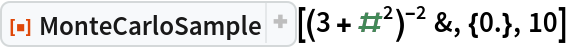Out=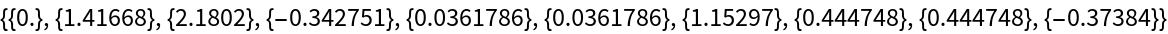Generate 1000 samples, discard the first 200 samples, and take every fifth sample:

 In:=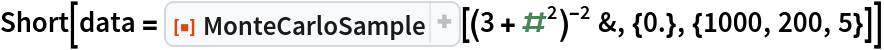Out=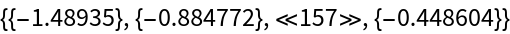In:=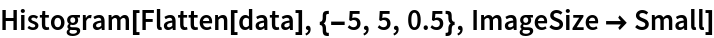Out=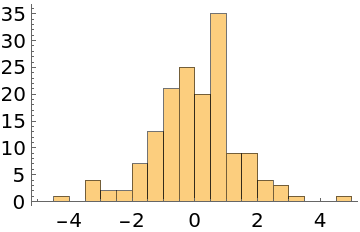Do automatic burn-in removal and thinning instead:

 In:=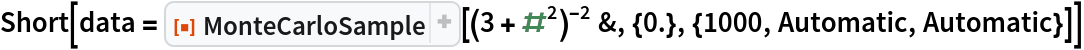Out=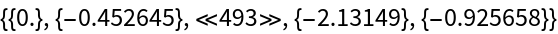In:=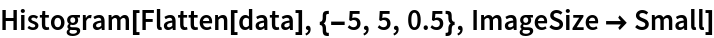Out=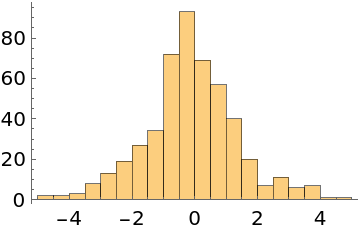Sample from a 2D normal distribution starting from an ensemble consisting of 10 random points:

 In:=Out=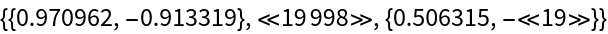In:=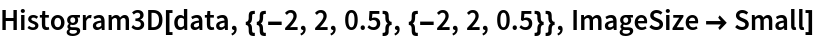Out=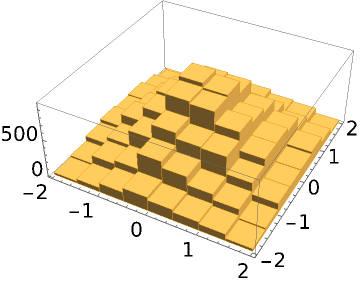Specify a prior in addition to the likelihood function:

 In:=Out=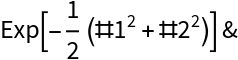Out=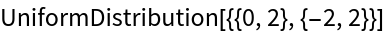In:=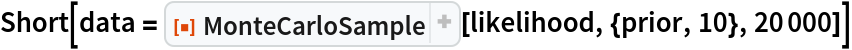Out=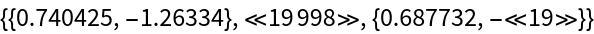In:=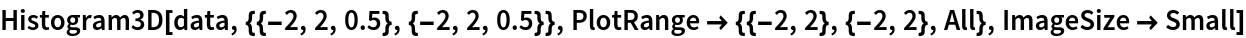Out=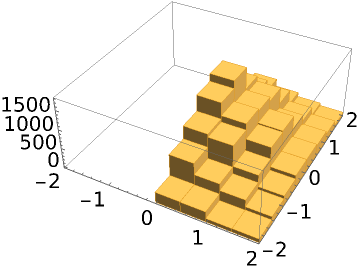### Options (8)

#### Method (3)

The random walk method takes jumps following a normal distribution by default. A custom distribution can also be specified:

 In:=Out=The adaptive Metropolis method takes jumps following an adaptive multinormal distribution that is derived from existing samples:

 In:=Out=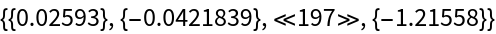In:=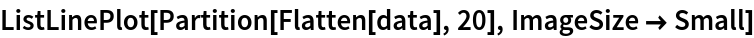Out=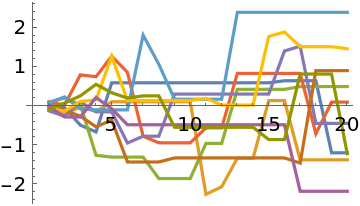The EMCEE and differential evolution methods take jumps being the displacement between two points multiplied by a scale factor. Increasing the number of initial points may improve the performance of these two methods:

 In:=Out=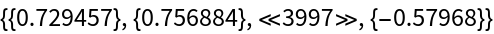In:=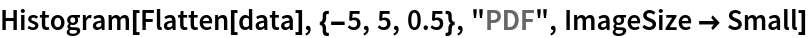Out=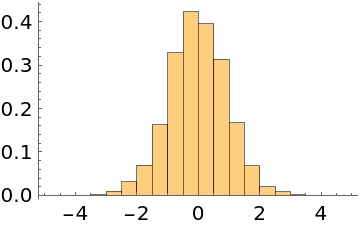#### LogProbability (2)

When the probability function evaluates to a tiny number, machine numbers may not be able to represent them accurately and the samples do not converge:

 In:=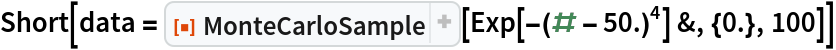Out=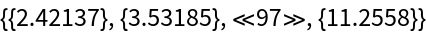In:=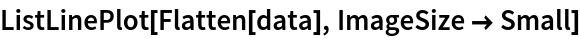Out=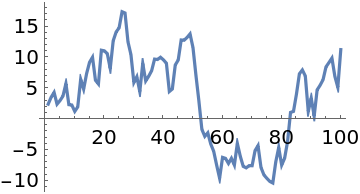"LogProbability"True indicates that the probability function is on a log scale, and the samples converge to x≈50:

 In:=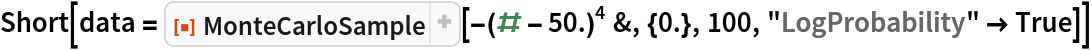Out=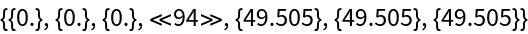In:=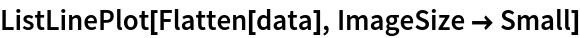Out=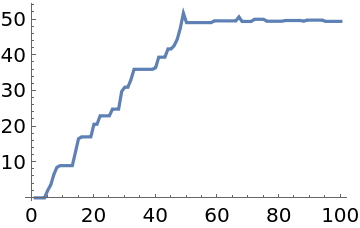#### TargetAcceptanceRate (1)

Setting the target acceptance rate is only effective for adaptive Metropolis or differential evolution methods. A higher acceptance rate decreases the step size and vice versa:

 In:=Out=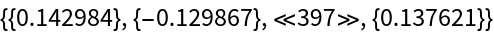In:=Out=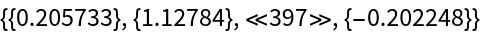In:=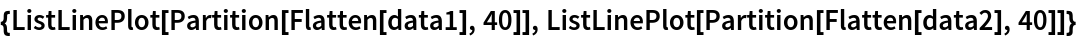Out=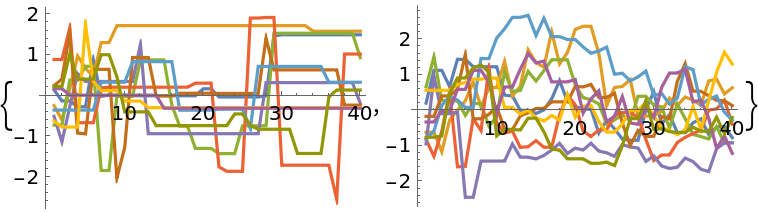#### ListableFunction (1)

If the probability function is listable, specifying "ListableFunction"True and employing a large number of initial points may significantly improve the performance:

 In:=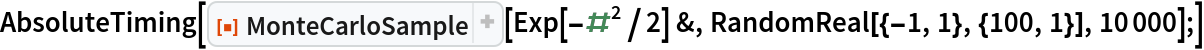Out=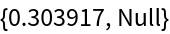In:=Out=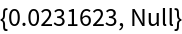#### Parallelization (1)

Enabling parallelization is only beneficial when the probability function can be run in parallel and each evaluation takes a long time:

 In:=Out=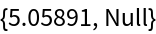In:=Out=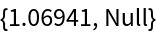### Applications (8)

#### Fit a distribution (4)

Get the posterior distribution of two parameters in a Student t distribution:

 In:=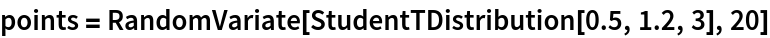Out=Choose a uniform prior over μ[-2,2], σ[0,3]:

 In:=Out=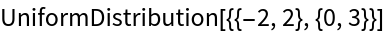Run MCMC:

 In:=Out=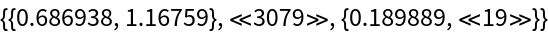In:=Out=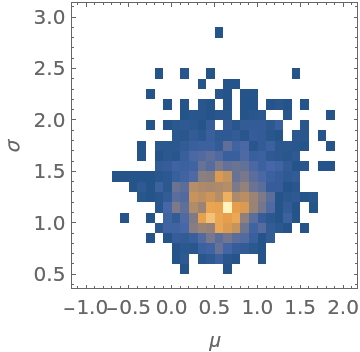The location of the peak in the posterior distribution matches the result given by EstimatedDistribution:

 In:=Out=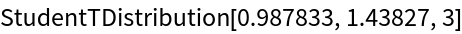#### Fit a sinusoidal function (4)

Get the posterior distribution of amplitude, frequency, phase, and mean of a sinusoidal function:

 In:=Out=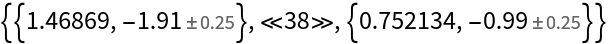In:=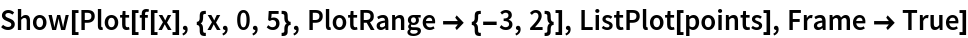Out=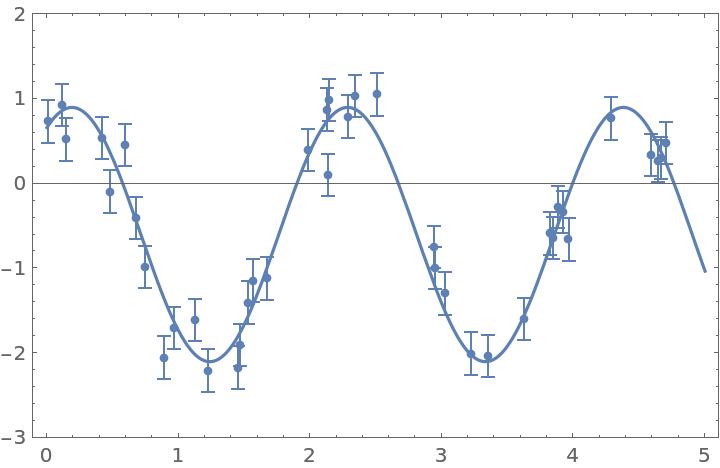The likelihood is derived based on the assumption that each point has a normally distributed error. Define the likelihood, and select the initial points for MCMC around the peak of the likelihood function:

 In:=Out=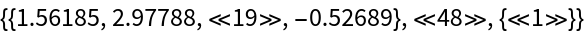Run MCMC:

 In:=Out=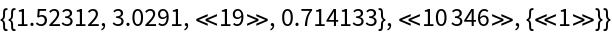The marginal distribution of all four parameters compared to their input values:

 In:=Out=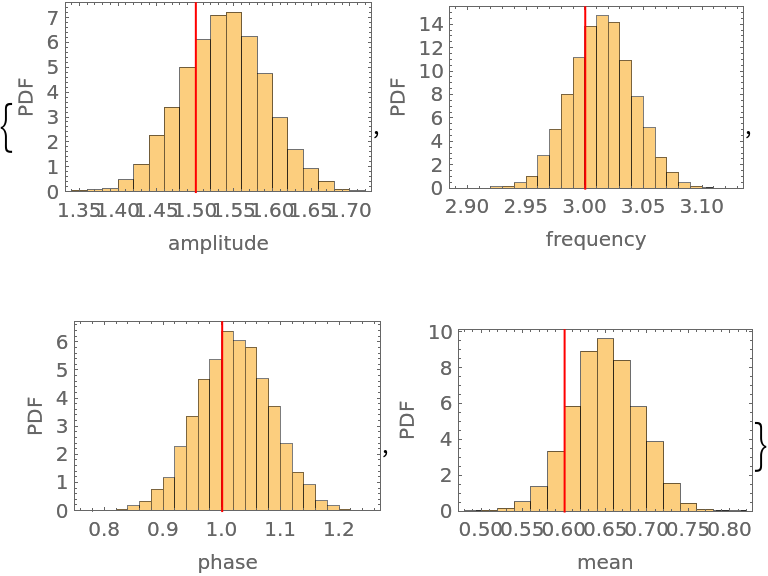### Properties and Relations (1)

The normalization of the probability function does not affect the result:

 In:=Out=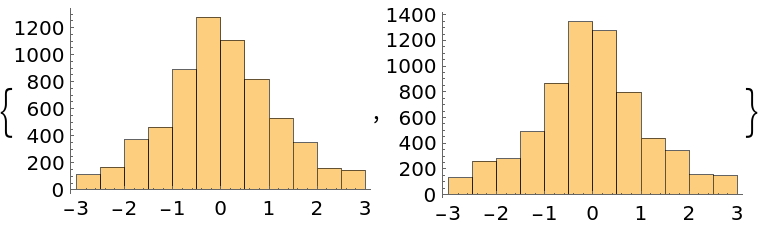### Possible Issues (2)

If all of the initial points are on an hyperplane, all samples will be on the same hyperplane when the EMCEE or differential evolution method is used:

 In:=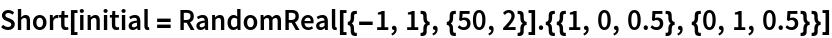Out=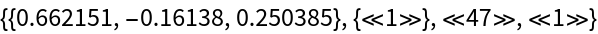In:=Out=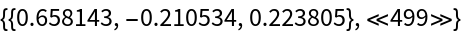In:=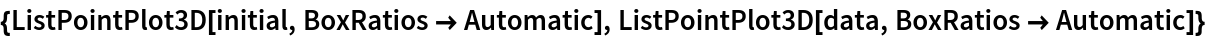Out=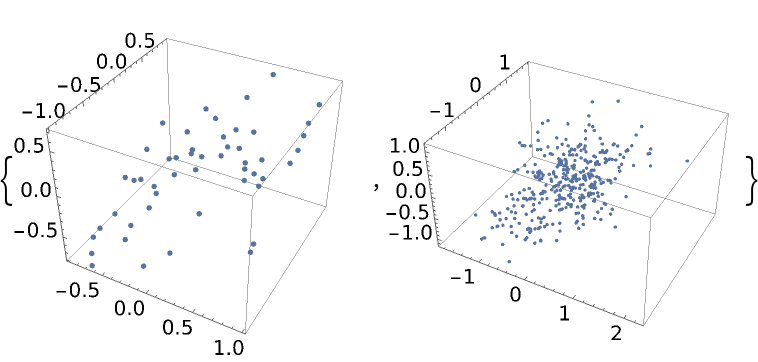Automatic burn-in removal is done by comparing the log-posteriors with the median log-posterior. In some cases, it may keep samples where thermalization has not been reached:

 In:=Out=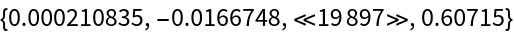In:=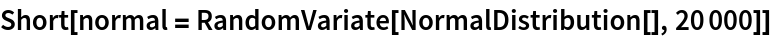Out=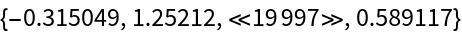In:=Out=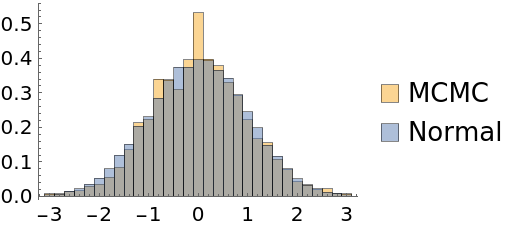To mitigate this problem, place the initial points on a wider range:

 In:=Out=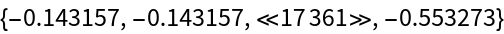In:=Out=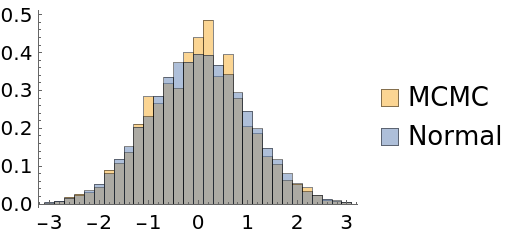Brian Lu

## Version History

• 1.0.0 – 05 July 2022